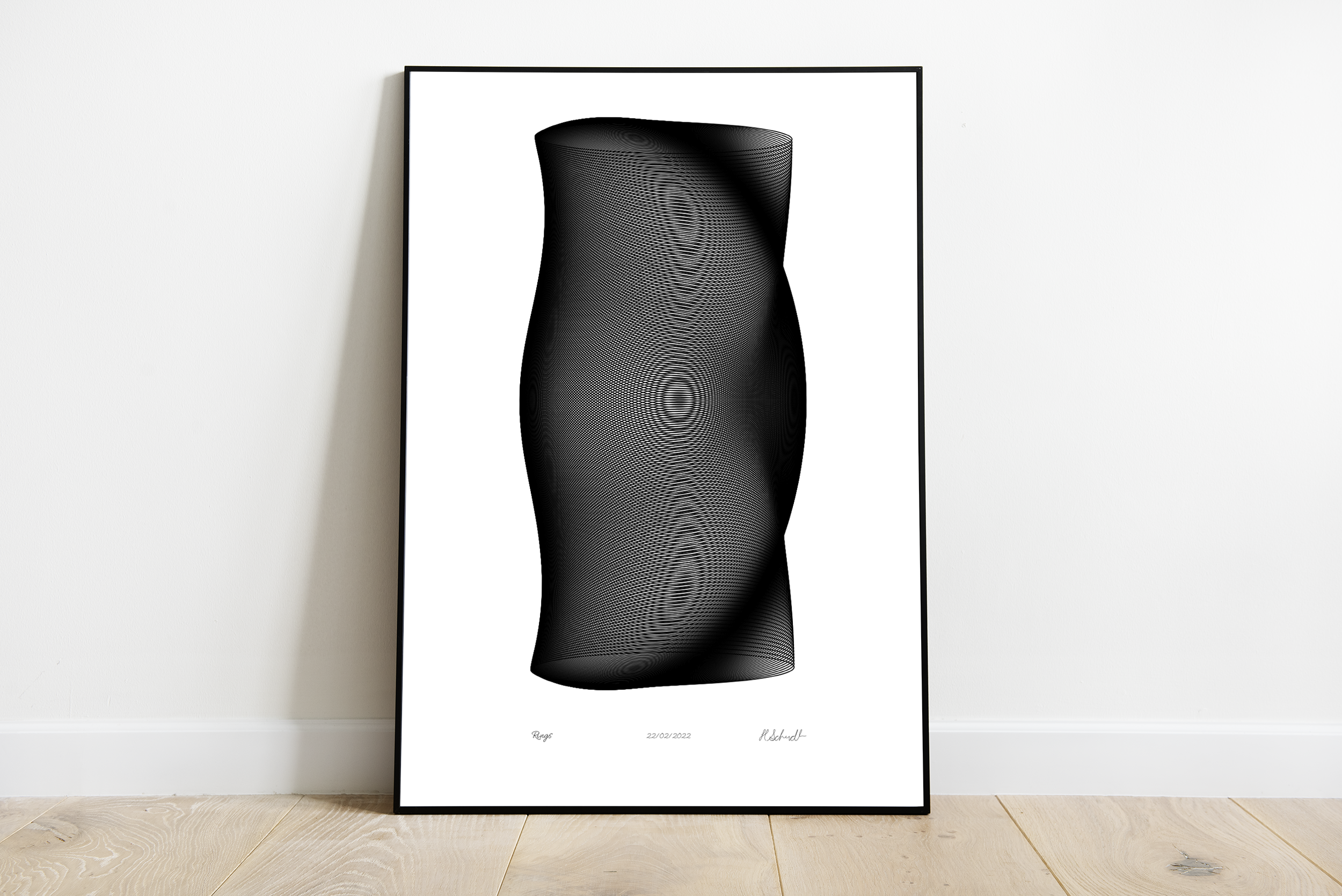# Rings (iteration 1), 2020When I started playing with the code for Rings I was just doodling a combination of ellipses and rotations. It was meant for the plotter from the beginning, so I stayed within my framework of lines and repetitions. I was done once I found the combination of both rotating and stretching the ellipses along the vertical axis.

``````  for (float j=0; j < 1; j++) {
float S = height/n/2;
float s = width * .35;
for (float i=1; i <= n; i++) {
pushMatrix();
float y = i * S;
translate(width/2, +y );
rotate(i/n* PI);
ellipse(0, 0, s, s*(.1 + pow(sin(i/n*HALF_PI*2), .85)) );
popMatrix();
}
s*=.95;
}``````